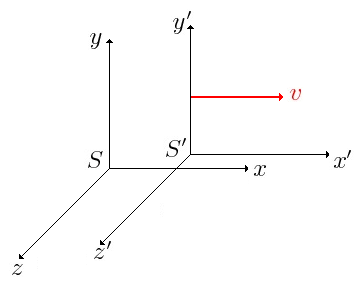iSoul In the beginning is reality.

# Two principles of velocity reciprocity

Velocity reciprocity in relativity theory is the relation between two observers, each associated with a frame of reference and moving at different, but constant, velocities. That is, an observer-frame S observes another observer-frame traveling with velocity +v relative to observer-frame S. A velocity reciprocity relation concerns the velocity of S that is observed by . Einstein’s principle of velocity reciprocity states that each velocity is the same magnitude (speed) but is in the opposite direction. That is, the velocity of S observed by  is –v.Einstein’s principle of velocity reciprocity reads

We postulate that the relation between the coordinates of the two systems is linear. Then the inverse transformation is also linear and the complete non-preference of the one or the other system demands that the transformation shall be identical with the original one, except for a change of v to −v. Ref.

This principle can be deduced from space–time homogeneity and (length) space isotropy of frames.

However, there is an alternate principle of velocity reciprocity that supports Galilean relativity. Consider the following scenario:Let’s say that an observer S on the platform pictured above observes an observer-frame moving with the train going to their left with speed v. If a left-hand convention is adopted, then the velocity of  in observer-frame S is positive: +v. Then how will an observer  in the train observe observer-frame S? An observer in observer-frame facing the station will observe observer-frame S receding to their left with the velocity v. If a left-hand convention is again adopted, the positive direction relative to an observer is to their left, and observer-frame will observe S traveling with a velocity +v going to their left.

Thus there are two different principles of velocity reciprocity: Einstein’s, in which the frames have the same orientation but their relative velocities are opposite, and the alternative, in which the frames have the opposite orientation (because they are facing one another) but their relative velocities are the same.

The difference between these two velocity reciprocity principles is in how orientation of an observer-frame is determined. Is the orientation of each observer-frame relative to itself, that is, the way an observer would face? Or is the orientation of each observer-frame relative to a common frame such as the Earth or the distant stars? Einstein’s principle of velocity reciprocity assumes that all reference frames have the same orientation. The alternative principle of velocity reciprocity assumes that all observer-frames are oriented by which way the observer is facing.